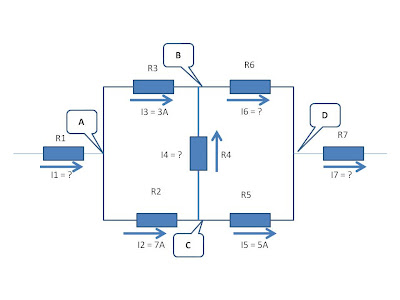## Wednesday, November 11, 2009

### Applications for DC Parallel Circuits

It's been a month and a half ago when I did an update on this blog. I've been busy with some things which I cannot explained to you right now. The reason why is that this project which is initiated by me is really intended for 2010. Although I'm doing a little update on this blog about Learning Electrical Engineering, the project still alive until such time I completed the course outline of this site.

I checked my stats awhile ago and I was surprised that my traffic still in tact with an average of 45 and above visitors a day. The number of subscribers is still increasing a bit compared when I started this blog. One thing you can expect from me, though I'm doing a little update on this blog, this site will still remain in search engines. I have now a PR2 from PR0. I would like to give thanks for those who supported and still visiting this site. Target keyword " learning electrical engineering " now dominates my rank in Google and Yahoo. Now let's continue of what I have left before.

The following illustrative problems below are just an applications of what I had presented on my post about DC Parallel Circuits.

From the previous post, I had illustrated to you the theory of the DC Parallel Circuits which consists of Part 1 and 2. Today, I will show you the application through problem solving.

Problem No. 1 : Let's begin solving parallel circuits in which I will show you how the current divides in each branch of circuits and how can we obtained the unknown values using Kirchhoff's Law. Suppose I have the circuit as shown in the diagram below. We need to find the unknown currents. We need to find the currents at I1, I4 , I6 and I7 . Click the image to enlarge.Obviously, we can find the current at junction A by simply performing the KCL or the Kirchoff's Current Law. We can see that the current divides at junction A. Therefore, I1 = I2 + I3 = 7A + 3 A= 10 amperes.

Taking a look at junction C. We can see that the current entering this junction I2 divides into I4 and I5 which becomes a total current to I2. Therefore we can say, I2 = I4 + I5, which becomes 7 A = I4 + 5 A, and since we are looking for I4 = 2 Amperes.

Now, we would like to solve for I6. Since, the flow of currents I3 and I4 are flowing toward junction B. We can say that, I6 = I3 + I4 = 3 A + 2 A = 5 Amperes.

The last requirement is I7. Since the currents I5 and I6 are flowing toward junction D. we can say that I7 = I5 + I6 = 5 A + 5A = 10 Amperes.

The example above I had shown you is how the currents divides in each branch. The next example will illustrate you the application of unequal resistors in parallel.

Problem No.2 : Three loads A, B and C are connected in parallel to a 230 volt source. Load A takes 9.2 KW, load B takes a current of 60 amperes and load C is a resistance of 4.6 ohms. Calculate (a) the resistance of loads A and B, (b) the total resistance of the three paralleled loads, (c) the total current, (d) the total power. Click the image to enlarge.Since we have different parameters given for each load, We have to solve first some missing requirements that we need in our calculations.

(a) Calculating Ra = Vt ^2 / Pa = 230 ^ 2 / 9,200 = 5.75 ohms
for Rb = Vt / Ib = 230 / 60 = 3.83 ohms
Note : The solutions shown above are just an applications of ohms law that we previously discussed.

(b) Calculating the total equivalent resistance of the circuits will follow:
Req = 1/ 1/5.75 + 1/3.83 + 1/4.6 = 1/ 0.174+ 0.261+0.217 = 1.53 ohms
Note : the formula used was just discussed on the previous post above DC PARALLEL CIRCUITS.

(c) Since we have already calculated the missing values, we can now solve for total current.
It = 230 / 1.53 = 150 Amperes

(d) To get the total power, since we already know the values of Vt and It. It will be now,
Pt = 230 x 150 = 34,500 watts or 34.5 KW.

For the above illustrative problem ( No.2 ) , I had shown you how the Ohms Law was also applied in solving the unknown quantities. This will surely applied when one value is missing. This is the best and basic technique that you can applied anytime you encountered such problems like this. The mentioned technique will also be applied when we reached complex AC circuitry.

This is the end of our basic circuitry in parallel connections. The next post will deal about series-parallel circuits lecture.

See you again on my next post.

Cheers!